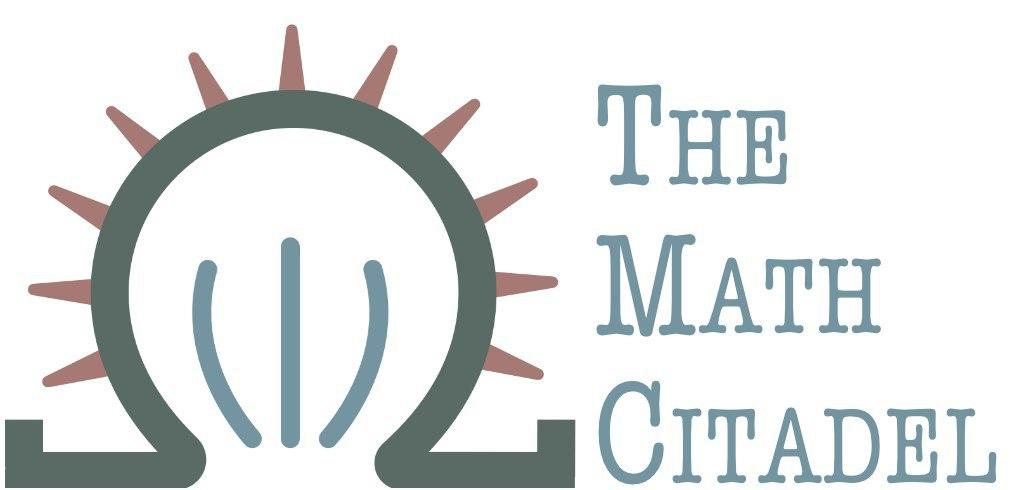## Defining an Anomaly

#### R. Traylor

Everyone speaks ../about anomaly detection and its importance, and for good reason. Excessive credit card charges can signal a stolen card. A large change in errors can signal an impending hard drive failure. Unusually low crop yields can signal a pest infestation.

In order to detect these strange occurrences, there are dozens of algorithms with plenty of data science buzzwords that attempt to solve this difficult problem; every company has one. Twitter has a “windowed seasonal variation on the standard ESD algorithm” that treats a data set a simply a collection of points. Others use “ARIMA forecasting” and then see if the most recent point lies “outside the confidence band for the forecast”. I can list a lot of other examples with plenty of acronyms and buzzwords that will make your head spin.

### You cannot detect what you cannot define

The mistake lies in trying to detect something that is not well-defined. Every one of those examples (and the dozens I didn’t list) are simply variations on the same mistake: putting the cart before the horse, where the cart is the distance measure from “normal” or “typical”, and the horse is “normal behavior”. In other words, we must define “normal”, before we can measure distance from it, and certainly before we can decide something is too far away from it.

Since we don’t define what is “normal” with any rigor, we cannot define “anomaly” with any certainty. As a result, the word “anomaly” has no mathematical definition. Let’s illustrate what I mean by a “mathematical definition”.

Take even and odd numbers, for example. An even number $n$ is defined as a natural number such that $n \bmod 2 \equiv 0$. (That is, $n$ is divisible by 2 with no remainder.) An odd number is defined as a natural number $m$ such that $m \bmod 2 \equiv 1$. (In other words, dividing $m$ by 2 leaves a remainder of 1.) Notice here that an odd number is not defined as “not an even number”; it is not defined relative to what an even number is. The definition stands alone. If I went crazy and changed the definition of “even number”, it wouldn’t affect the definition of an odd number.

### Negative of a negative is not always a positive

The problem with defining an “anomaly” as “not normal” is the same as defining an odd number as “not even”. In fact, most attempts at a definition for an anomaly are: “a point that is too far away from the rest of the data” or “an excessively large deviation from the mean”, or “a point that lies outside the median +/- 1.5 IQR”1.

All of these definitions are vague and, more importantly, relative to something else. Just like with the definitions of “even” and “odd” numbers, a definition has to be able to stand on its own, and not rely on something that can shift or be subject to someone’s judgment.

By defining an anomaly as relative to the data, or the center of the data, or the like, any attempts at detecting them start off on shaky ground. Datasets are a moving target; every time you sample (or draw balls from urns, or measure the heights of 42 randomly chosen American men, or flip 10 coins), the starting point for anomaly detection changes.

In mathematics, we call the observed data realizations of a random variable or a random process. The outcome of a game of roulette is a random variable. Each time you play, you observe an instance of that variable (and either win or lose money), but each spin of the wheel is going to result in a different outcome.

The current debates all center on the type of distance metric to use to decide how far away from “normal” you are, or the threshold for how far is “too far”. But the only glimpse we have of “normal” is an ever-changing set of realizations of a random variable. All these debates and algorithms are ignoring the the fundamental issue: defining the problem. The working definition of an anomaly is shaky and relies on someone else’s judgment, and thus the argument over anomaly detection becomes one of opinion, and not how to implement rigorous mathematical principles that data science rests on.

Uncertainty has to be accounted for. When you draw a data set, the mean and the standard deviation require estimation, because neither are known in advance. Thus, we must account for the additional uncertainty in estimating two parameters, rather than just one. Thus, we risk compounding errors when the very definition of what we seek to detect is not firm.

Detecting strange instances in data is an important problem, but we want to avoid adding another algorithm to the pile. In the next article, we will examine the mathematical topic of intervention analysis and discuss why we should think ../about “weird data points” in terms of interventions rather than anomalies.